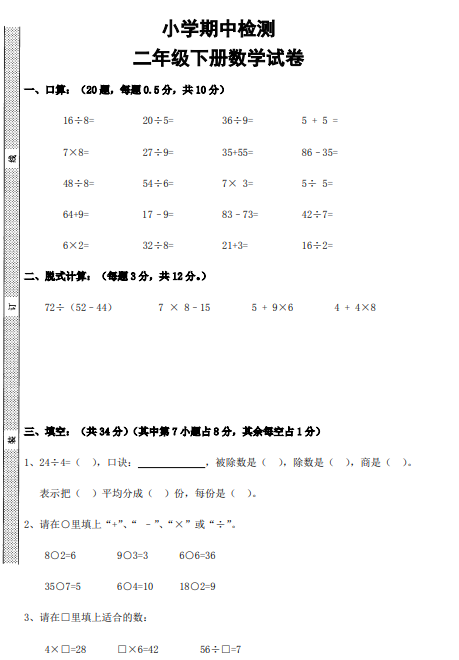16÷8= 20÷5= 36÷9= 5 + 5 =
7×8= 27÷9= 35+55= 86–35=
48÷8= 54÷6= 7× 3= 5÷ 5=
64+9= 17–9= 83–73= 42÷7=
6×2= 32÷8= 21+3= 16÷2=##### 评论信息